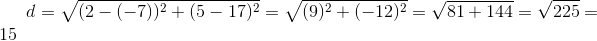ISEE Lower Level Math : Lines

Example Questions

2 Next →

Example Question #403 : Geometry

How long is a line segment on the number line if it goes fromto?Explanation:

To find the length of a line on the number line when one point is negative and the other is positive, you must the the distances of both from zero.isspaces from zero where is the other point is just.  So the distance is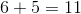.

Example Question #404 : Geometry

What is the distance between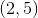and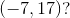The distance formula is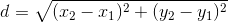.
Let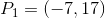and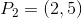: